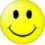# AMC10/12A

Who took the AMC10/12??? Did you meet your expectations?

Scores are not yet out, so please tell me if they are out :)

Thank YouNote by Bob Yang
7 years, 5 months ago

This discussion board is a place to discuss our Daily Challenges and the math and science related to those challenges. Explanations are more than just a solution — they should explain the steps and thinking strategies that you used to obtain the solution. Comments should further the discussion of math and science.

When posting on Brilliant:

• Use the emojis to react to an explanation, whether you're congratulating a job well done , or just really confused .
• Ask specific questions about the challenge or the steps in somebody's explanation. Well-posed questions can add a lot to the discussion, but posting "I don't understand!" doesn't help anyone.
• Try to contribute something new to the discussion, whether it is an extension, generalization or other idea related to the challenge.

MarkdownAppears as
*italics* or _italics_ italics
**bold** or __bold__ bold
- bulleted- list
• bulleted
• list
1. numbered2. list
1. numbered
2. list
Note: you must add a full line of space before and after lists for them to show up correctly
paragraph 1paragraph 2

paragraph 1

paragraph 2

[example link](https://brilliant.org)example link
> This is a quote
This is a quote
    # I indented these lines
# 4 spaces, and now they show
# up as a code block.

print "hello world"
# I indented these lines
# 4 spaces, and now they show
# up as a code block.

print "hello world"
MathAppears as
Remember to wrap math in $$ ... $$ or $ ... $ to ensure proper formatting.
2 \times 3 $2 \times 3$
2^{34} $2^{34}$
a_{i-1} $a_{i-1}$
\frac{2}{3} $\frac{2}{3}$
\sqrt{2} $\sqrt{2}$
\sum_{i=1}^3 $\sum_{i=1}^3$
\sin \theta $\sin \theta$
\boxed{123} $\boxed{123}$

Sort by:

You can find the answer key online with a google search. I exceeded my expectations! The test had beautiful problems, like #25, (which I skipped)

- 7 years, 5 months ago

Oh Thanks :)

- 7 years, 5 months ago

The AIME cutoff's going to have to be 120 this year.

- 7 years, 5 months ago

I'm mad at myself for making FOUR stupid mistakes:

1. Forgot to add the original 35 miles (#11)

2. For the palindrome question, I added all $abc$ rather than $abcba$

3. For the arith/geo sequence question, forgot $a

4. For question 24 with the absolute values (which was stupidly easy and didn't deserve to be question 24) I thought $f_0 (x)$ was $f_1 (x)$!

But it's okay. I'm taking the 12B next week too. (I got a predicted 102 on this one, sadly)

Favorite problems: Question 21 and 23.

Question 21 because it's one of those "looks hard but isn't" questions

Question 23 because it's a great question.

Solution 23: Note that $\frac{1}{99} = .\overline{01} = (\frac{1}{100} + \frac{1}{10000} + \dots$, so $\frac{1}{99^2} = (\frac{1}{100} + \frac{1}{10000} + \frac{1}{1000000} + \dots) (\frac{1}{100} + \frac{1}{10000} + \frac{1}{1000000} + \dots)$.

Multiplying the first term, $\frac{1}{100}$, by the whole of $(\frac{1}{100} + \frac{1}{10000} + \frac{1}{1000000} + \dots)$. ) yields the repeating decimal $0.00\overline{01}$

Multiplying the second term yields $0.0000\overline{01}$, and so forth. Thus, the decimal looks like so:

$0.000010203040506070809101112131415\dots969799$ -- note that $98 + 99 + 100$ at the end turns into $990000$.

Adding it up, the sum of the first $9$ natural numbers occurs $10$ times, so that's $450$. Then the digit $1-9$ appears as the "tens place" $10$ times each, so that's another $450$. Subtract $8 + 9 = 17$ since $98$ isn't counted to get $883$ as the answer. $\blacksquare$

- 7 years, 5 months ago

i tooko the 10A, and i got a 114. Not as good as I wished it could be, but I am overall satisfied

@Michael tong- you're 23, how you take the amc12

- 7 years, 5 months ago

read my profile -- "I'm 17, not 23!"

- 7 years, 5 months ago

AMC12....expecting a 118.5, enough to make the AIME. During the test, I only focused on the first 20 questions(as I mentioned in my strategy), I skipped number 8 on due to the confusing wording of the problem, later I found out all of other participants in my school was able to do that problem. Next there was number 18, which twisted up my brain......

Other than these, I feel satisfied since I didn't leave any counting and geometry problems blank :)

- 7 years, 5 months ago

I got a 132, but I made a stupid mistake on number 4. It was really easy this time; previously, I had scored 1.5 less than the cutoff almost every time.

- 7 years, 5 months ago

On the AMC 10, I did my modular arithmetic wrong and got #2 wrong...

- 7 years, 5 months ago

lol same here :P

- 7 years, 5 months ago

I feel dumb... Made a dumb arithmetic mistake on #17 [36+9=42] and ended up with 112.5

- 7 years, 5 months ago

I got 130.5 on AMC 10. Made a stupid mistake on number 4 (multiplied by 2). Finished the first 22, missed one. I heard from my friend that #23 was easy, but otherwise, I think I did well. The AMC answers are all on AoPS, so you can go on there to check. Well, I'm taking the AMC 12B next week. Hopefully, I can get at least a 110.

- 7 years, 5 months ago